Test: Logic Gates & Boolean Algebra - 2

# Test: Logic Gates & Boolean Algebra - 2

Test Description

## 10 Questions MCQ Test Topicwise Question Bank for Electronics Engineering | Test: Logic Gates & Boolean Algebra - 2

Test: Logic Gates & Boolean Algebra - 2 for Electronics and Communication Engineering (ECE) 2023 is part of Topicwise Question Bank for Electronics Engineering preparation. The Test: Logic Gates & Boolean Algebra - 2 questions and answers have been prepared according to the Electronics and Communication Engineering (ECE) exam syllabus.The Test: Logic Gates & Boolean Algebra - 2 MCQs are made for Electronics and Communication Engineering (ECE) 2023 Exam. Find important definitions, questions, notes, meanings, examples, exercises, MCQs and online tests for Test: Logic Gates & Boolean Algebra - 2 below.
Solutions of Test: Logic Gates & Boolean Algebra - 2 questions in English are available as part of our Topicwise Question Bank for Electronics Engineering for Electronics and Communication Engineering (ECE) & Test: Logic Gates & Boolean Algebra - 2 solutions in Hindi for Topicwise Question Bank for Electronics Engineering course. Download more important topics, notes, lectures and mock test series for Electronics and Communication Engineering (ECE) Exam by signing up for free. Attempt Test: Logic Gates & Boolean Algebra - 2 | 10 questions in 30 minutes | Mock test for Electronics and Communication Engineering (ECE) preparation | Free important questions MCQ to study Topicwise Question Bank for Electronics Engineering for Electronics and Communication Engineering (ECE) Exam | Download free PDF with solutions
 1 Crore+ students have signed up on EduRev. Have you?
*Multiple options can be correct
Test: Logic Gates & Boolean Algebra - 2 - Question 1

### Select the Boolean function(s) equivalent to x + yz, where x, y, and z are Boolean variables, and + denotes logical OR operation.

Detailed Solution for Test: Logic Gates & Boolean Algebra - 2 - Question 1

Concept:
Boolean function:

The variables used in Boolean Algebra only have one of two possible values, a logic “0” and a logic “1” but an expression can have an infinite number of variables all labeled individually to represent inputs to the expression.
For example, variables A, B, C, etc, give us a logical expression of A + B = C, but each variable can ONLY be a 0 or a 1.
The given expression is = x + yz the equivalent expression is,
Option 1: x + z + xy
= x + z + xy
= x(1+y)+ z  (∴ 1 + x = x + 1 = x)
= x + z it is not equivalent x + yz.
Option 2: (x + y)(x + z)
= (x + y)(x + z)
= x + xz + yx + yz
= x(1+ z+ y) + yz  (∴1 + x = x + 1 = x)
= x + yz it is equivalent x + yz.
Option 3: x + xy + yz
= x + xy + yz
= x(1 + y) +yz
= x + yz  it is equivalent x + yz.
Option 4: x + xz + xy
= x + xz + xy
= x(1+z+y) (∴1 + x = x + 1 = x)
= x it is not equivalent x + yz.
Hence the correct answer is option 2 and option 3.

Test: Logic Gates & Boolean Algebra - 2 - Question 2

### Given below are two statements: Statement I: A two-input OR gate and a two-input AND gate have similar inputs. but outputs may be the same or different. Statement II: An AND gate has inputs A and B. The input B is always low, the state of input A can affect the output. In the light of the above statements, choose the correct answer from the options given below:

Detailed Solution for Test: Logic Gates & Boolean Algebra - 2 - Question 2

Concept:
A two-input OR gate shows the output as: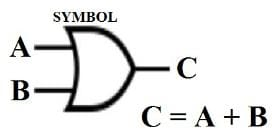A two-input AND gate shows the output as: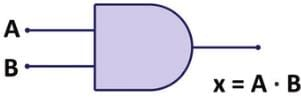Circuit symbol of AND gate

Calculations:
Given:
Statement I:
A two-input OR gate and a two-input AND gate have similar inputs.
For OR gate output = A + B, AND gate output is = A.B
So, statement 1 is incorrect.
The output of AND gate is shown by its truth table: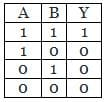According to Statement II: An AND gate has inputs A and B. The input B is always low,
Then, B shows 0 as input then any Value of A can not change the output. So, this statement is incorrect.
Hence, Option (2) is correct that both statements are incorrect.

Test: Logic Gates & Boolean Algebra - 2 - Question 3

### Four statements are given below. Identify the correct statement.

Detailed Solution for Test: Logic Gates & Boolean Algebra - 2 - Question 3
• AND, OR, NOT gates are the basic gates.
• The logic gates which are derived from the basic gates like AND, OR, NOT gates are known as derived gates. NAND, NOR, XOR, and XNOR are the derived gates.
• A universal gate is a gate which can implement any Boolean function without need to use any other gate type. The NAND and NOR gates are universal gates.
Test: Logic Gates & Boolean Algebra - 2 - Question 4

Write the simplified equation for the given K-Map.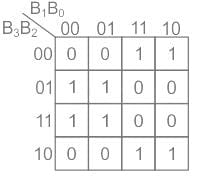Detailed Solution for Test: Logic Gates & Boolean Algebra - 2 - Question 4

Concept:
K-map:

• K-map (Karnaugh Map) is a pictorial method used to minimize Boolean expression without having to use Boolean Algebra theorems and equation manipulation.
• K-map can be thought of as a special version of a truth table.
• Using K-map, expression with two to four variables are easily minimized.
• K-maps are also referred to as 2D truth tables as each K-map is nothing but a different format of representing the values present in a one-dimensional truth table.
• To simplify a logic expression with two inputs, we require a K-map with 4 cells (= 22)
• Similarly, a logic expression with four inputs we require a K-map with 16 cells (= 24)
• Each cell within K-map has a definite place value which is obtained by using on encoding technique known as Gray code.
• For n-variable K-map, with 2n cells, try to group 2n cells first, then for 2n-1 cells, next for 2n-2 cells, and so on until the group contains only 2° cells ie. Isolated bits (if any)
• Also remember, the number of cells in a group must be equal to an integer power to 2 i.e. 1, 2, 4, 8, ...

Calculation: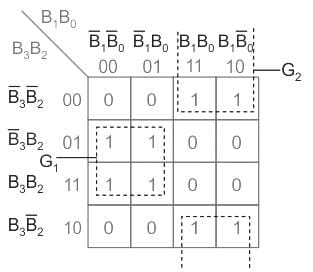→ There are no 16 bits group, no 8-bits group, but there are 2-four bits group
→ Eliminate the variables for which the corresponding hit appears within the group as both 0 and 1.

• Group 1 → B̅1 B2
• Group 2 → B1 B̅2

→ Therefore in SOP form (sum of products) output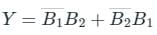Test: Logic Gates & Boolean Algebra - 2 - Question 5

In Boolean algebra, (A.A̅) + A = ?

Detailed Solution for Test: Logic Gates & Boolean Algebra - 2 - Question 5

(A.A̅) + A
= 0 + A = A
All Boolean algebra laws are shown below: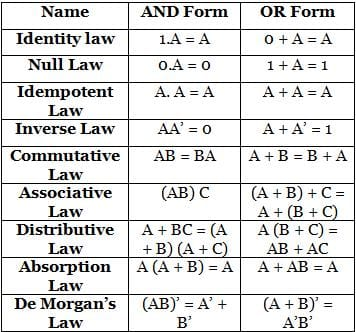Test: Logic Gates & Boolean Algebra - 2 - Question 6

The basic gates are:

Detailed Solution for Test: Logic Gates & Boolean Algebra - 2 - Question 6
• AND, OR, NOT gates are the basic gates.
• The logic gates which are derived from the basic gates like AND, OR, NOT gates are known as derived gates. NAND, NOR, XOR, and XNOR are the derived gates.
• A universal gate is a gate which can implement any Boolean function without need to use any other gate type. The NAND and NOR gates are universal gates.
Test: Logic Gates & Boolean Algebra - 2 - Question 7

The output Y of the logic circuit given below is:-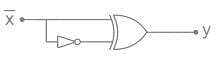Detailed Solution for Test: Logic Gates & Boolean Algebra - 2 - Question 7

XOR GATE
Symbol: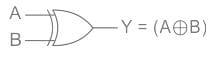Truth Table: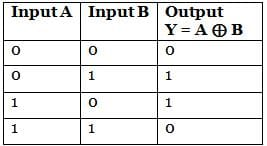Output Equation: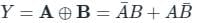Key Points:

1) If B is always High, the output is the inverted value of the other input A, i.e. A̅.
1) The output is low when both the inputs are the same.
2) The output is high when both the inputs are different.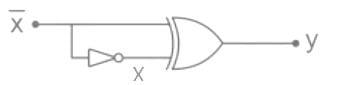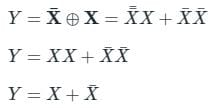Y = 1

Test: Logic Gates & Boolean Algebra - 2 - Question 8

Which one of the following gives the simplified sum of products expression for the Boolean function F = m0 + m2 + m3 + m5, where m0, m2, m3 and m5 are minterms corresponding to the inputs A, B and C and A as the MSB and C as the LSB?

Detailed Solution for Test: Logic Gates & Boolean Algebra - 2 - Question 8

F = ∑m (0, 2, 3, 5)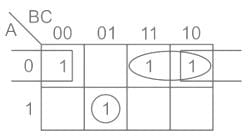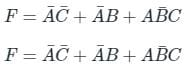Test: Logic Gates & Boolean Algebra - 2 - Question 9

The complement of the expression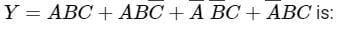Detailed Solution for Test: Logic Gates & Boolean Algebra - 2 - Question 9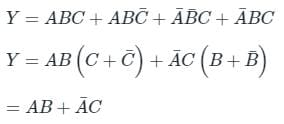To find the complement of Y.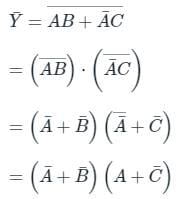Test: Logic Gates & Boolean Algebra - 2 - Question 10

Simplification of the function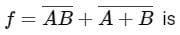Detailed Solution for Test: Logic Gates & Boolean Algebra - 2 - Question 10

Concept:
De Morgan’s law states that: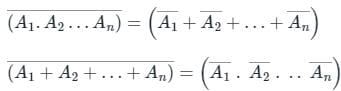Application: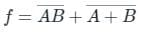This can be written as:
f = A̅ + B̅ + A̅ B̅
f = A̅ (1 + B̅) + B̅
f = A̅ + B̅
Again using De-Morgan's property, we get: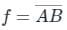## Topicwise Question Bank for Electronics Engineering

193 tests
Information about Test: Logic Gates & Boolean Algebra - 2 Page
In this test you can find the Exam questions for Test: Logic Gates & Boolean Algebra - 2 solved & explained in the simplest way possible. Besides giving Questions and answers for Test: Logic Gates & Boolean Algebra - 2, EduRev gives you an ample number of Online tests for practice

## Topicwise Question Bank for Electronics Engineering

193 tests(Scan QR code)